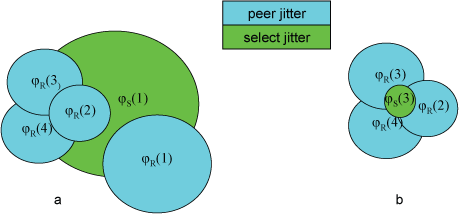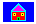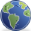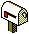The clock cluster algorithm processes the truechimers produced by the clock select algorithm to produce a list of survivors. These survivors are used by the mitigation algorithms to discipline the system clock. The cluster algorithm operates in a series of rounds, where at each round the truechimer furthest from the offset centroid is pruned from the population. The rounds are continued until a specified termination condition is met. This page discusses the algorithm in detail.

First, the truechimer associations are saved on an unordered list with each candidate entry identified with index i (i = 1, …​, n), where n is the number of candidates. Let θ(i), be the offset and λ(i) be the root distance of the ith entry. Recall that the root distance is equal to the root dispersion plus half the root delay. For the ith candidate on the list, a statistic called the select jitter relative to the ith candidate is calculated as follows. Let

di(j) = |θ(j) − θ(i)| λ(i),

where θ(i) is the peer offset of the ith entry and θ(j) is the peer offset of the jth entry, both produced by the clock filter algorithm. The metric used by the cluster algorithm is the select jitter φS(i) computed as the root mean square (RMS) of the di(j) as j ranges from 1 to n. For the purpose of notation in the example to follow, let φR(i) be the peer jitter computed by the clock filter algorithm for the ith candidate.

The object at each round is to prune the entry with the largest metric until the termination condition is met. Note that the select jitter must be recomputed at each round, but the peer jitter does not change. At each round the remaining entries on the list represent the survivors of that round. If the candidate to be pruned is preemptable and the number of candidates is greater than the maxclock threshold, the association is demobilized. This is useful in the schemes described on the Automatic Server Discovery Schemes page. The maxclock threshold default is 10, but it can be changed using the `maxclock` option of the `tos` command. Further pruning is subject to the following termination conditions, but no associations will be automatically demobilized.

The termination condition has two parts. First, if the number of survivors is not greater than the minclock threshold set by the `minclock` option of the `tos` command, the pruning process terminates. The minclock default is 3, but can be changed to fit special conditions, as described on the Mitigation Rules and the prefer Keyword page.Figure 1. Cluster Algorithm

The second termination condition is more intricate. Figure 1 shows a round where a candidate of (a) is pruned to yield the candidates of (b). Let φmax be the maximum select jitter and φmin be the minimum peer jitter over all candidates on the list. In (a), candidate 1 has the highest select jitter, so φmax = φS(1). Candidate 4 has the lowest peer jitter, so φmin = φR(4). Since φmax > φmin, select jitter dominates peer jitter, the algorithm prunes candidate 1. In (b), φmax = φS(3) and φminR(4). Since φmax < φmin, pruning additional candidates does not reduce select jitter, the algorithm terminates with candidates 2, 3 and 4 as survivors.

The survivor list is passed on to the mitigation algorithms, which combine the survivors, select a system peer, and compute the system statistics passed on to dependent clients. Note the use of root distance λ as a weight factor at each round in the clock cluster algorithm. This is to favor the survivors with the lowest root distance and thus the smallest maximum error.Home PageSite MapContacts